# Analyzing Obesity in England With Python

I saw a sign at the gym yesterday that said, “Children are getting fatter every decade”. Below that sign stood a graph that basically showed that in five years the average English child will weigh as much as a tractor.

I found this claim slightly unbelievable, so I decided to investigate…

## The Data#

The data is taken from Data.gov.uk. We’ll be using the 2014 XLS file. Download it, and open it in your spreadsheet tool of choice.

Then navigate to sheet 7.2, as it contains the data we are looking for: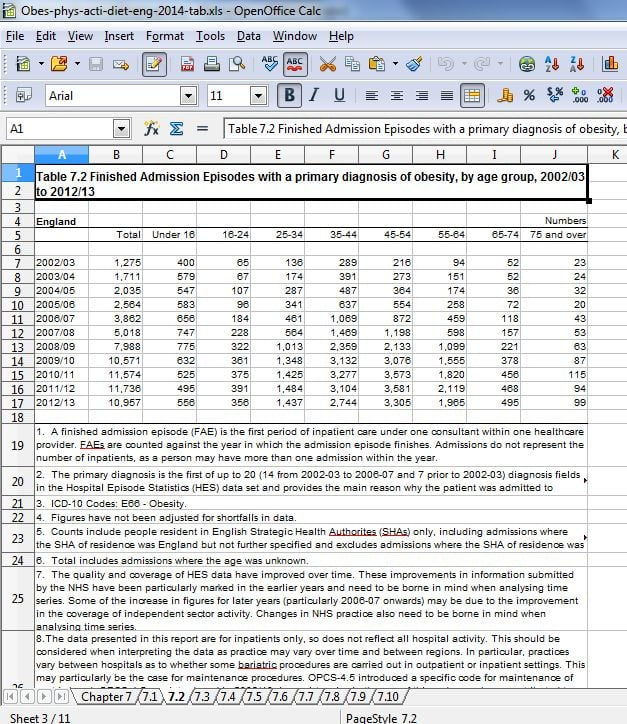Now, before we jump into analyzing the data with Pandas, let’s take a step back and address the elephant in the room: If you can perform the analysis/plotting in Excel, why would you use Python?

## Python vs Excel#

Should I use Python or Excel?

This question is often asked by people just starting out in data analysis. While Python may be popular with the programming community, Excel is much more prevalent in the wider world. Most officer managers, sales people, marketers, etc. use Excel - and there’s nothing wrong with that. It’s a great tool if you know how to use it well, and it has turned many non-technical people into expert analysts.

The answer to whether you should use Python or Excel is not an easy one to answer. But in the end, there is no either/or: Instead, you can use them together.

Excel is great for viewing data, performing basic analysis, and drawing simple graphs, but it really isn’t suitable for cleaning up data (unless you are willing to dive into VBA). If you have a 500MB Excel file with missing data, dates in different formats, no headers, it will take you forever to clean it by hand. The same can be said if your data is spread across a dozen CSV files, which is fairly common.

Doing all that cleanup is trivial with Python and Pandas, a Python library for data analysis. Built on top of Numpy, Pandas makes high-level tasks easy, and you can write your results back to an Excel file, so you can continue to share the results of your analysis with non-programmers.

So while Excel isn’t going away, Python is a great tool if you want clean data and perform higher-level data analysis.

## The Code#

Right, let’s get started with the code - which you can grab from the project repo along with the spreadsheet I linked to above so you don’t have to download it again.

Start by creating a new script called obesity.py and import Pandas as well as matplotlib so that we can plot graphs later on:

``````import pandas as pd
import matplotlib.pyplot as plt
``````

Make sure you install both dependencies: `pip install pandas matplotlib`

Next, let’s read in the Excel file:

``````data = pd.ExcelFile("Obes-phys-acti-diet-eng-2014-tab.xls")
``````

And that’s it. In a single line we read in the entire Excel file.

Let’s print what we have:

``````print data.sheet_names
``````

Run the script.

``````\$ python obesity.py
[u'Chapter 7', u'7.1', u'7.2', u'7.3', u'7.4', u'7.5', u'7.6', u'7.7', u'7.8', u'7.9', u'7.10']
``````

Look familiar? These are the sheets we saw earlier. Remember, we will be focusing on sheet 7.2. Now if you look at 7.2 in Excel, you will see that the top 4 rows and bottom 14 rows contain useless info. Let me rephrase: It’s useful for humans, but not for our script. We only need rows 5-18.

## Cleanup#

So when we read the sheet, we need to make sure any unnecessary info is left out.

``````# Read 2nd section, by age
data_age = data.parse(u'7.2', skiprows=4, skipfooter=14)
print data_age
``````

Run again.

``````   Unnamed: 0  Total  Under 16  16-24  25-34  35-44  45-54  55-64  65-74  \
0         NaN    NaN       NaN    NaN    NaN    NaN    NaN    NaN    NaN
1     2002/03   1275       400     65    136    289    216     94     52
2     2003/04   1711       579     67    174    391    273    151     52
3     2004/05   2035       547    107    287    487    364    174     36
4     2005/06   2564       583     96    341    637    554    258     72

#...snip...#
``````

We read the sheet, skipping the top 4 rows as well as the bottom 14 (as they contain data not useful to us). We then printed what we have. (For simplicity, I’m only showing the first few lines of the printout.)

The first line represents the column headers. Right off the bat you can see Pandas is quite smart since it picked up most of the headers correctly. Except for the first one, of course - e.g., `Unnamed: 0`. Why is that? Simple. Look at the file in Excel, and you see that it is missing a header for the year.

Another problem is that we have an empty line in the original file, and that is showing up as `NaN` (Not a number).

So we now need to do two things:

1. Rename the first header to `Year`, and
2. Get rid of any empty rows.
``````# Rename unamed to year
data_age.rename(columns={u'Unnamed: 0': u'Year'}, inplace=True)
``````

Here we told Pandas to rename the column Unnamed: 0 to Year. using the in-built function `rename()`.

`inplace = True` modifies the existing object. Without this, Pandas will create a new object and return that.

Next let’s drop the empty rows filled with `NaN`:

``````# Drop empties
data_age.dropna(inplace=True)
``````

There is one more thing we need to do that will make our lives easier. If you look at the data_age table, the first value is a number. This is the index, and Pandas uses the default Excel practice of having a number as the index. However, we want to change the index to Year. This will make plotting much easier, since the index is usually plotted as the x axis.

``````data_age.set_index('Year', inplace=True)
``````

We set the index to `Year`.

Now print our cleaned up data:

``````print "After Clean up:"
print data_age
``````

And run:

``````         Total  Under 16  16-24  25-34  35-44  45-54  55-64  65-74  \
Year
2002/03   1275       400     65    136    289    216     94     52
2003/04   1711       579     67    174    391    273    151     52
2004/05   2035       547    107    287    487    364    174     36
2005/06   2564       583     96    341    637    554    258     72
#...snip...#
``````

Much better. You can see the index is now the `Year`, and all `NaN`s are gone.

## Charts#

Now we can plot what we have.

``````# Plot
data_age.plot()
plt.show()
``````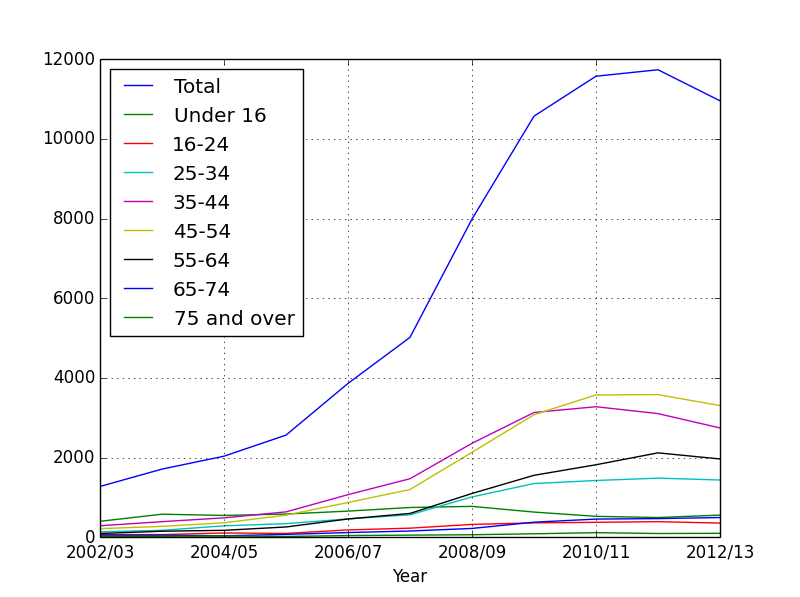Oops. There is a problem: Our original data contains a total field that is overshadowing everything else. We need to get rid of it.

``````# Drop the total column and plot
data_age_minus_total = data_age.drop('Total', axis=1)
``````

`axis =1` is slightly confusing, but all it really means is - drop the columns, as described from this Stack Overflow question.

Let’s plot what we have now.

``````data_age_minus_total.plot()
plt.show()
``````

Much better. We can actually see individual age groups now. Can you see which age group has the highest obesity?Coming back to our original question: Are children getting fatter?

Let’s just plot a small section of the data: children under the age of 16 and grown ups in the age range of 35-44.

``````plt.close()

data_age['Under 16'].plot(label="Under 16")
data_age['35-44'].plot(label="35-44")
plt.legend(loc="upper right")
plt.show()
``````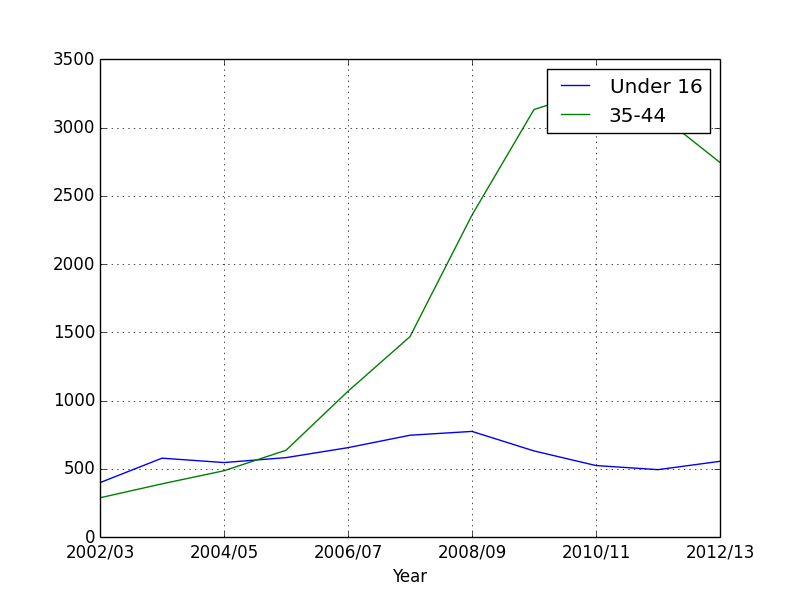## So who is getting fatter?#

Right. What do we see?

While children’s obesity has gone slightly down, their parents have ballooned. So it seems the parents need to worry about themselves rather than their children.

## But what about the future?#

The graph still doesn’t tell us what will happen to children’s obesity in the future. There are ways to extrapolate graphs like these into the future, but I must give a warning before we proceed: The obesity data has no underlying mathematical foundation. That is, we can’t find a formula that will predict how these values will change in the future. Everything is essentially guesswork. With this warning in mind, let’s see how we can try to extrapolate our graph.

First, Scipy does provide a function for extrapolation, but it only works for monotically increasing data (while our data goes up and down).

We can try curve fitting:

• Curve Fitting tries to fit a curve through points on a graph, by trying to generate a mathematical function for the data. The function may or may not be very accurate, depending on the data.
• Polynomial Interpolation Once you have an equation, you can use polynomial interpolation to try and interpolate any value on the graph.

We’ll use these two functions together to try and predict the future for England’s children:

``````kids_values = data_age['Under 16'].values
x_axis = range(len(kids_values))
``````

Here, we extract the values for children under 16. For the x axis, the original graph had dates. To simplify our graph, we’ll just be using the the numbers 0-10.

Output:

``````array([ 400.,  579.,  547.,  583.,  656.,  747.,  775.,  632.,  525., 495.,  556.])
[0, 1, 2, 3, 4, 5, 6, 7, 8, 9, 10]
``````

One more thing: Curve fitting uses different degrees of polynomials. In very simple terms,the higher the degree, the more accurate the curve fitting will be, but there is also the chance that the results will be garbage. Scipy will sometimes warn you if the degree is too high. Don’t worry, this will be more clear when we look at some examples.

``````poly_degree = 3
curve_fit = np.polyfit(x_axis, kids_values, poly_degree)
poly_interp = np.poly1d(curve_fit)
``````

We set the polynomial degree to 3. We then use the Numpy `polyfit()` function to try to fit a graph through the data we have. The `poly1d()` function is then called on the equation we generated to create a function that will be used to generate our values. This returns a function called `poly_interp` that we will use below:

``````poly_fit_values = []

for i in range(len(x_axis)):
poly_fit_values.append(poly_interp(i))
``````

We loop from 0 to 10, and call the `poly_interp()` function on each value. Remember, this is the function we generated when we ran the curve fitting algorithm.

Before moving on, let’s see what the different polynomial degrees mean.

We will plot both the original data, and our own data, to see how close our equation reached the ideal data:

``````plt.plot(x_axis, poly_fit_values, "-r", label = "Fitted")
plt.plot(x_axis, kids_values, "-b", label = "Orig")

plt.legend(loc="upper right")
``````

The original data will be plotted in blue and labelled Orig, while the generated data will be red and labelled Fitted.

With a polynomial value of 3: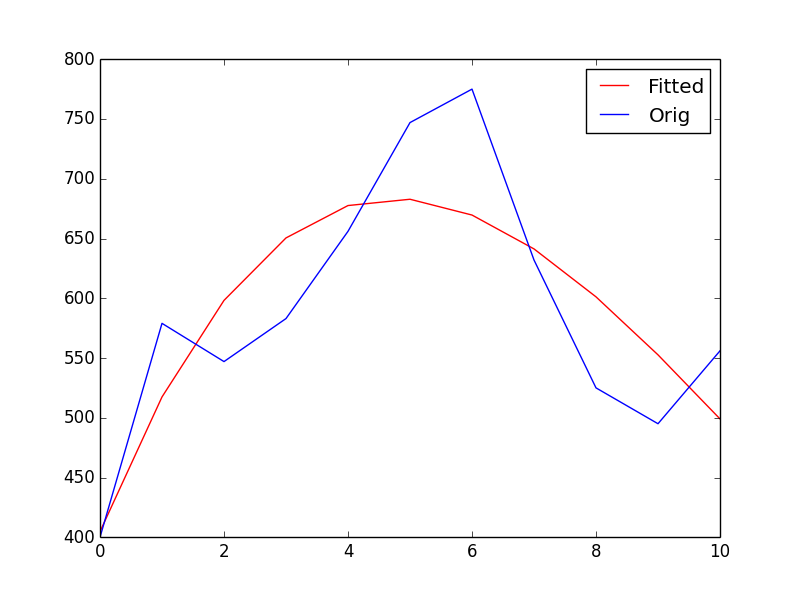We see it isn’t that good a fit, so let’s try 5: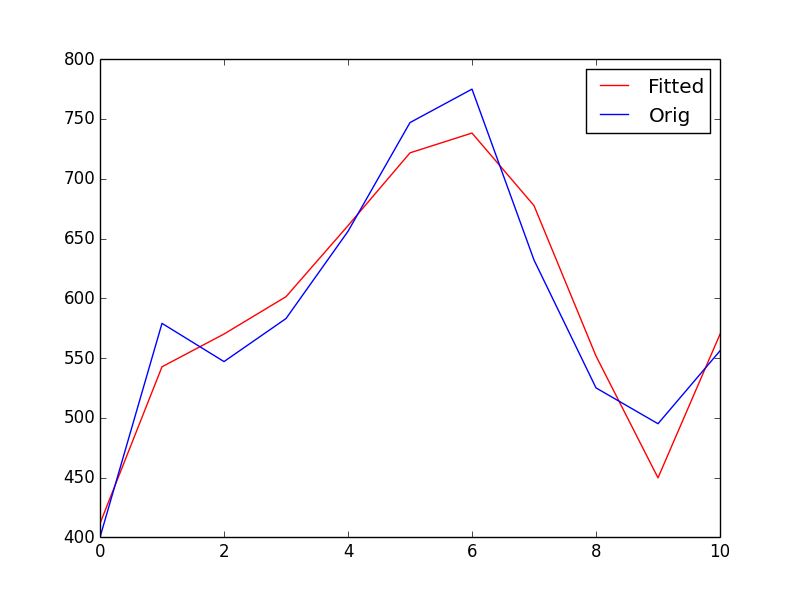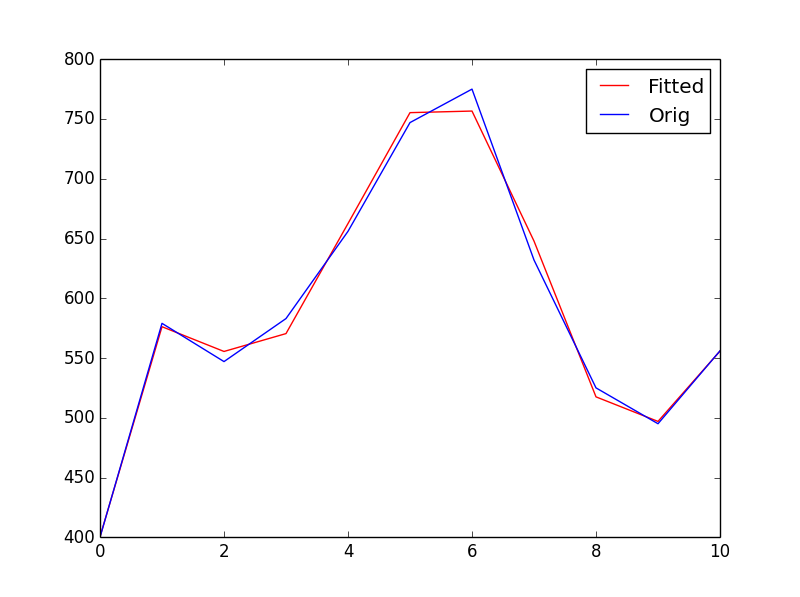Now we get an almost perfect match. So, why wouldn’t we always use higher values?

Because the higher values have been so tightly coupled to this graph, they make prediction useless. If we try to extrapolate from the graph above, we get garbage values. Trying different values, I found that polynomial degrees of 3 and 4 were the only ones that give accurate results, so that’s what we’ll be using.

We are going to re-run our `poly_interp()` function, this time for values from 0-15, to predict five years into the future.

``````x_axis2 = range(15)

poly_fit_values = []
for i in range(len(x_axis2)):
poly_fit_values.append(poly_interp(i))
``````

This is the same code as before. Let’s again see the results with polynomial degrees of 3 and 4. The new extrapolated line is the green one and shows our prediction.

With 3: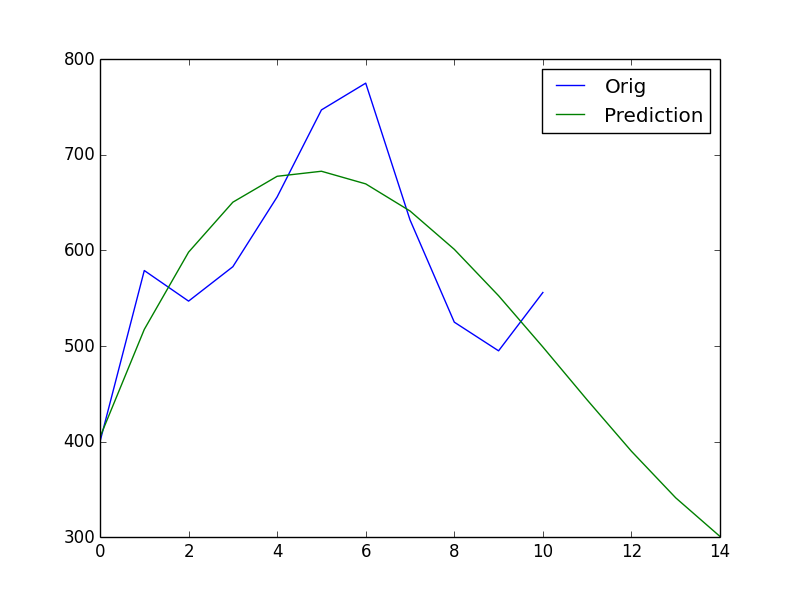Here, the obesity is going down. How about 4?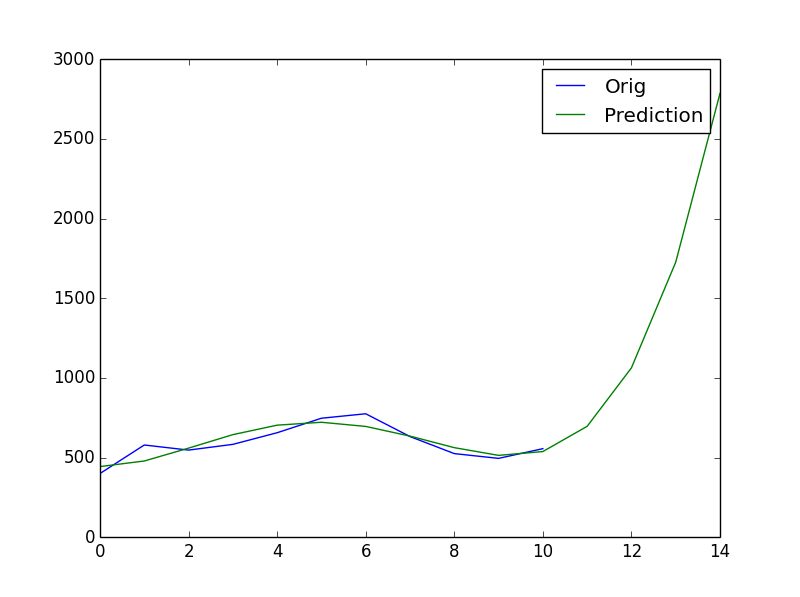But here, it is shooting up, so kids will end up weighing like tractors!

Which of the two graphs is correct? It depends on whether you are working for the government or the opposition.

This is actually a feature, not bug. You must have heard these political debates where two sides draw the exact opposite conclusions from the same data? Now you see how it’s possible to draw radically different conclusions by tweaking small parameters.

And that is the reason we must be careful when accepting figures and graphs from lobbyists, especially if they are not willing to share the raw data. Sometimes, predictions are better left to astrologers.

Cheers!

🐍 Python Tricks 💌

Get a short & sweet Python Trick delivered to your inbox every couple of days. No spam ever. Unsubscribe any time. Curated by the Real Python team.Shantnu has worked in the low level/embedded domain for ten years. Shantnu suffered at the hands of C/C++ for several years before he discovered Python, and it felt like a breath of fresh air.